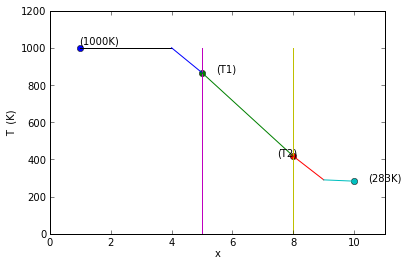# Chapter1:Fundamental Concepts¶

### Example 1.1 Page No.7¶

In :
k=9.4              # thermal conductivity in [BTU/hr.ft. ˚Rankine]
q=6.3              # heat flux in [BTU/s. sq.ft]
T1=350             # the outside surface temperature of one aide of the wall [˚F]

Q=6.3*3600         # [BTU/hr.sq.ft]
dx=0.5             # thickness in [inch]
Dx=0.5/12.0          # thickness in [ft]
T2=T1-(Q*Dx/k)     # [˚F]

print"The required temperature on the other side of the firewall is ",round(T2,1),"F"

The required temperature on the other side of the firewall is  249.5 F


### Example 1.2 Page No.9¶

In :
k_ss=14.4    #  thermal conductivity of stainless steel in [W/m.K]
dt_ss=40     # [K]
dt_al=8.65   # [K]
dz_ss=1      # [cm]
dz_al=3      # [cm]

k_al=k_ss*dt_ss*dz_al/(dt_al*dz_ss);# thermal conductivity of Al in [W/m.K]

print"The thermal conductivity of aluminium is",round(k_al,0),"W/m.K"

The thermal conductivity of aluminium is 200.0 W/m.K


### Example 1.3 Page No.13¶

In :
h_c=3               # convective coefficient in [BTU/hr.ft**2
A=30*18             # Cross sectional area in ft**2
T_w=140             # Roof surface temperature in degree Fahrenheit
T_inf=85           # Ambient temperature in degree Fahrenheit

dT= (T_w-T_inf)
Q_c=h_c*A*dT       # Convective heat transfer in BTU/hr

print"The heat transferred by convection is",round(Q_c,2),"BTU/hr"

The heat transferred by convection is 89100.0 BTU/hr


### Example 1.4 Page No.14¶

In :
D=0.0243            # diameter in meter
L=0.2             # length in meter
A=3.14*D*L           # cross-sectional area in sq.m
cp=4200.0              # specific heat of water in J/kg.K
T_b2=21.4             # temperature of bulk fluid in degree celsius
T_in=20.0             # temperature of inlet water in degree celsius
T_w=75.0              # temperature of wall in degree celsius
Q=500.0              # volumetric flow rate in cc/s
density=1000       # density of water in kg/cu.m

m=Q*density/10**6  # mass flowa rate in kg/s
hc=m*cp*(T_b2-T_in)/(A*(T_w-T_in))

print"The average film conductance is ",round(hc,0),"W/sq.m. K"

The average film conductance is  3503.0 W/sq.m. K


### Example 1.5 Page No.18¶

In :
W=14         # width in ft
L=30.0       # length in ft
A=W*L        # area in ft**2
F_12=1       # view factor assumed to be 1
T1=120+460   # driveway surface temperature  in degree Rankine
T2=0        # space temperature assumed to be 0 degree Rankine

sigma=0.1714*10**(-8)   # value of Stefan-Boltzmann's constant in BTU/(hr.ft**2.(degree Rankine)**4)
e=0.9       # surface emissivity
q=sigma*A*e*F_12*((T1)**4-(T2)**4);

print"The heat loss rate by radiation is ",round(q,0),"BTU/hr"

The heat loss rate by radiation is  73319.0 BTU/hr


### Example 1.6 Page No.19¶

In :
A=420.0              # area in sq.ft
T1=580.0          # driveway surface temperature in degree Rankine
T2=0               # surface temperature assumed to be 0 degree Rankine
Qr=73320             # heat loss rate in BTU/hr

hr=Qr/(A*(T1-T2))  # radiation thermal conductance in BTU/(hr.ft**2.(degree Rankine)

print"the radiation thermal conductance is  ",round(hr,2),"BTU/(hr. sq.ft R)"

the radiation thermal conductance is   0.3 BTU/(hr. sq.ft R)


### Example 1.7 Page No. 21¶

In [ ]:
%matplotlib inline

In :
A=1.0                # assuming A=1 m**2 for convenience
hc1_avg=15.0         # taking average of extreme values for hc [W/m**2.K]
k=(0.38+0.52)/2.0    # thermal conductivity of common brick in W/M.k
L=0.1                #10 cm converted into m
Rk=(L/(k*A))         # resistance of construction material, assume common brick

T_inf1=1000.0 # temperature of exhaust gases in K
T_inf2=283.0 # temperature of ambient air in K
Rcl=1/(hc1_avg*A)   # resistance on left side of wall [K/W]
Rc2=Rcl
q=(T_inf1-T_inf2)/(Rcl+Rk+Rc2)  # heat transferred per unit area
T_in=T_inf1-Rcl*q    #inlet temprature
T_out=T_inf2+Rc2*q

print"(b)"
print"The resistance on left side of wall is ",round(Rcl,2),"K/W"
print"The resistance of construction material of wall is",round(Rk,2),"K/W"
print"The resistance on right side of wall is ",round(Rc2,2),"K/W"
print"(c)The Heat transferred per unit area is ",round(q/1000,2),"kw"
print "(d)"
print"The inside wall temperature is ",round(T_in,0),"K"
print"The outside wall temperature is",round(T_out,1),"K"

import matplotlib.pyplot as plt
fig = plt.figure()

x1=[5,5]
T1=[0,1000]

x2=[8,8]
T2=[0,1000]

x3=[1,4]
T3=[1000,1000]

x4=[4,5]
T4=[1000,866]

x5=[5,8]
T5=[866,417]

x6=[8,9]
T6=[417,290]

x7=[9,10]
T7=[290,283]

xlabel("x")
ylabel("T  (K)")
plt.xlim((0,11))
plt.ylim((0,1200))

ax.plot(, , 'o')
ax.annotate('(1000K)', xy=(1,1020))

ax.plot(, , 'o')
ax.annotate('(T1)', xy=(5.5,866))

ax.plot(, , 'o')
ax.annotate('(T2)', xy=(7.5,417))
ax.plot(, , 'o')
ax.annotate('(283K)', xy=(10.5,283))

a1=plot(x1,T1)
a2=plot(x2,T2)
a3=plot(x3,T3)
a4=plot(x4,T4)
a5=plot(x5,T5)
a6=plot(x6,T6)
a7=plot(x7,T7)
show(a1)

(b)
The resistance on left side of wall is  0.07 K/W
The resistance of construction material of wall is 0.22 K/W
The resistance on right side of wall is  0.07 K/W
(c)The Heat transferred per unit area is  2.02 kw
(d)
The inside wall temperature is  866.0 K
The outside wall temperature is 417.4 K### Example 1.8 Page No.24¶

In :
k=0.604        # [BTU/(hr.ft.degree Rankine)]
hc=3.0          # average value for natural convection in BTU/(hr.ft**2.degree Rankine)
ew=0.93
f_wr=1.0         # shape factor
sigma= 0.1714*10**(-8) # BTU/(hr.ft**2.degree Rankine).
L=4/12.0        # length in ft
T1=80+460       # temperature of side-walk in degree Rankine
T_inf=20+460    # temperature of ambient air in degree Rankine
T_r=0           # assuming space temperature to be 0 degree Rankine

a=((k/L)+hc)       #Coefficient of Tw in the equation
b=(sigma*ew*f_wr)  #Coefficient of Tw**4 in the equation
c=(k*T1/L)+(hc*T_inf)+(sigma*f_wr*ew*T_r**4)  #right hans side of the equation
Tw1=470            #assumed first value of temprature
LHS1=a*Tw1+b*Tw1**4
Tw2=480            #assumed 2nd value of temprature
LHS2=a*Tw2+b*Tw2**4
Tw3=490            #assumed 3rd value of temprature
LHS3=a*Tw3+b*Tw3**4
Tw4=485            #assumed 4th value of temprature
LHS4=a*Tw4+b*Tw4**4
Tw5=484.5            #assumed fifth value of temprature
LHS5=a*Tw5+b*Tw5**4

print"RHS",round(c,1)
print"LHS at surface Temprature 1=",round(LHS1,1)
print"LHS at surface Temprature 2=",round(LHS2,1)
print"LHS at surface Temprature 3=",round(LHS3,0)
print"LHS at surface Temprature 4=",round(LHS4,1)
print"LHS at surface Temprature 5=",round(LHS5,1)
print"\nLHS is close enough to RHS at Temprature 484.5. So Surface Temprature is",Tw5,"R"

RHS 2418.5
LHS at surface Temprature 1= 2339.4
LHS at surface Temprature 2= 2394.4
LHS at surface Temprature 3= 2450.0
LHS at surface Temprature 4= 2422.0
LHS at surface Temprature 5= 2419.2

LHS is close enough to RHS at Temprature 484.5. So Surface Temprature is 484.5 R

In [ ]: